Courses

# Compound Stresses In Machine Parts Mechanical Engineering Notes | EduRev

## Mechanical Engineering : Compound Stresses In Machine Parts Mechanical Engineering Notes | EduRev

The document Compound Stresses In Machine Parts Mechanical Engineering Notes | EduRev is a part of the Mechanical Engineering Course Machine Design.
All you need of Mechanical Engineering at this link: Mechanical Engineering

Introduction

The elements of a force system acting at a section of a member are axial force, shear force and bending moment and the formulae for these force systems were derived based on the assumption that only a single force element is acting at the section. Figure-2.2.1.1 shows a simply supported beam while figure-2.2.1.2 shows the forces and the moment acting at any cross-section X-X of the beam. The force system can be given as:

Axial force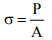Bending moment :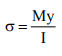Shearforce :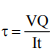Torque :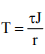where, σ is the normal stress, τ the shear stress, P the normal load, A the crosssectional area, M the moment acting at section X-X, V the shear stress acting at section X-X, Q the first moment of area, I the moment of inertia, t the width at which transverse shear is calculated, J the polar moment of inertia and r the radius of the circular cross-section.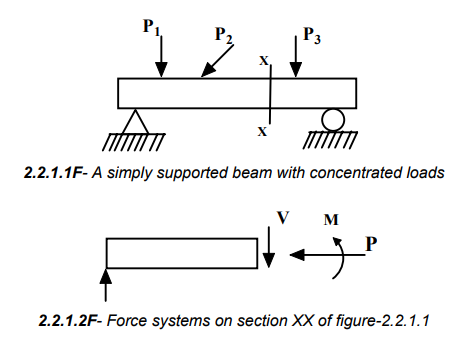Combined effect of these elements at a section may be obtained by the method of superposition provided that the following limitations are tolerated: (a) Deformation is small (figure-2.2.1.3)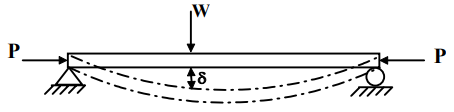If the deflection is large, another additional moment of Pδ would be developed. (b) Superposition of strains are more fundamental than stress superposition and the principle applies to both elastic and inelastic cases.

Strain superposition due to combined effect of axial force P and bending moment M.

Figure-2.2.2.1 shows the combined action of a tensile axial force and bending moment on a beam with a circular cross-section. At any cross-section of the beam, the axial force produces an axial strain εa while the moment M causes a

bending strain. If the applied moment causes upward bending such that the strain at the upper most layer is compressive (-ε2) and that at the lower most layer is tensile (+ε1), consequently the strains at the lowermost fibre are additive (εa+ε1) and the strains at the uppermost fibre are subtractive (εa2). This is demonstrated in figure-2.2.2.1.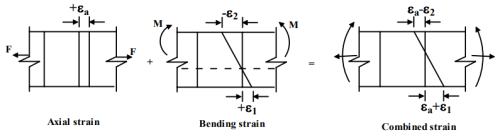Superposition of stresses due to axial force and bending moment

In linear elasticity, stresses of same kind may be superposed in homogeneous and isotropic materials. One such example (figure-2.2.3.1) is a simply supported beam with a central vertical load P and an axial compressive load F. At any section a compressive stress of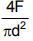and a bending stress of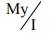are produced. Here d is the diameter of the circular bar, I the second moment of area and the moment is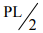where the beam length is 2L. Total stresses at the upper and lower most fibres in any beam cross-section are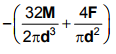And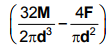respectively. This is illustrated in figure-2.2.3.2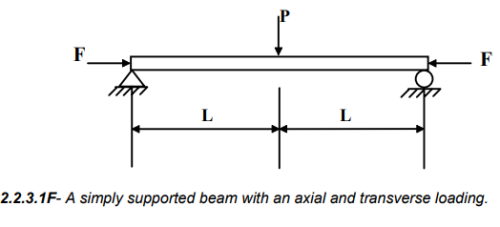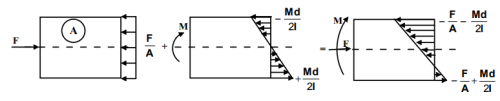2.2.3.2F- Combined stresses due to axial loading and bending moment.

Superposition of stresses due to axial force, bending moment and torsion

Until now, we have been discussing the methods of compounding stresses of same kind for example, axial and bending stresses both of which are normal stresses. However, in many cases members on machine elements are subjected to both normal and shear stresses, for example, a shaft subjected to torsion, bending and axial force. This is shown in figure-2.2.4.1. A typical example of this type of loading is seen in a ship’s propeller shafts. Figure-2.2.4.2 gives a schematic view of a propulsion system. In such cases normal and shearing stresses need to be compounded.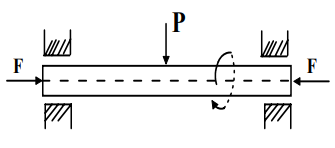2.2.4.1F- A simply supported shaft subjected to axial force bending moment and torsion.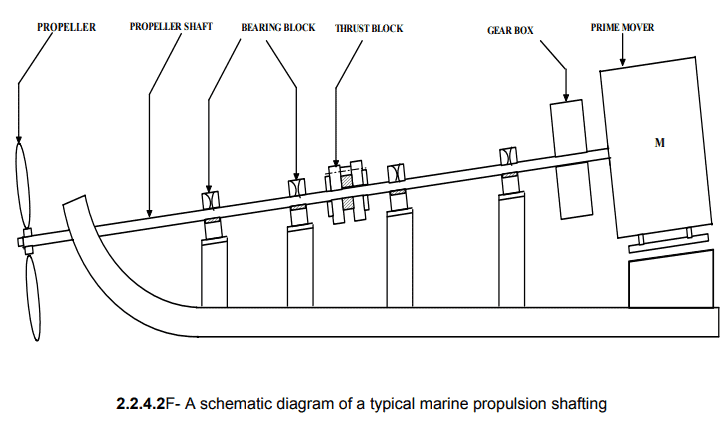Transformation of plane stresses

Consider a state of general plane stress in x-y co-ordinate system. We now wish to transform this to another stress system in, say, x′- y′ co-ordinates, which is inclined at an angle θ. This is shown in figure-2.2.5.1.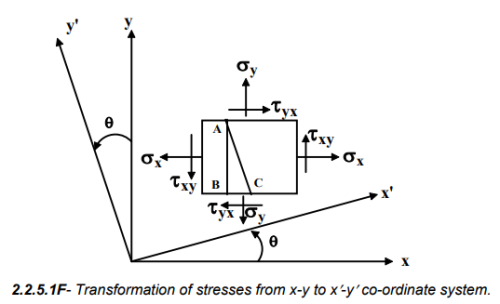A two dimensional stress field acting on the faces of a cubic element is shown in figure-2.2.5.2. In plane stress assumptions, the non-zero stresses are σx, σy and τxyyx.We may now isolate an element ABC such that the plane AC is inclined at an angle θ and the stresses on the inclined face are σ′x and τ′xy .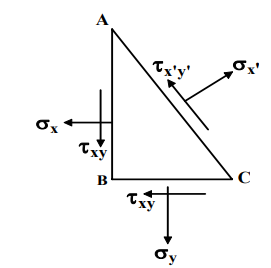2.2.5.2F- Stresses on an isolated triangular element

Considering the force equilibrium in x-direction we may write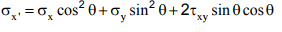This may be reduced to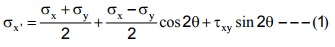Similarly, force equilibrium in y-direction gives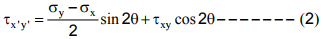Since plane AC can assume any arbitrary inclination, a stationary value of σx′ is given by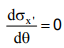This gives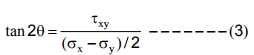This equation has two roots and let the two values of θ be θ1 and (θ1+90). Therefore these two planes are the planes of maximum and minimum normal stresses. Now if we set  τx'y' 0 we get the values of θ corresponding to planes of zero shear stress.

This also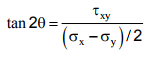gives

And this is same as equation (3) indicating that at the planes of maximum and minimum stresses no shearing stress occurs. These planes are known as Principal planes and stresses acting on these planes are known as Principal stresses. From equation (1) and (3) the principal stresses are given as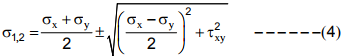In the same way, condition for maximum shear stress is obtained from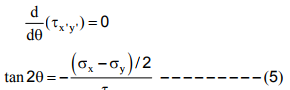This also gives two values of θ say θ2 and (θ2+90o ), at which shear stress is maximum or minimum. Combining equations (2) and (5) the two values of maximum shear stresses are given by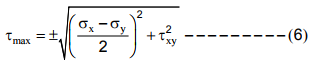One important thing to note here is that values of tan2θ2 is negative reciprocal of tan2θ1 and thus θ1 and θ2 are 45o apart. This means that principal planes and planes of maximum shear stresses are 45o apart. It also follows that although no shear stress exists at the principal planes, normal stresses may act at the planes of maximum shear stresses.

An example

Consider an element with the following stress system (figure-2.2.6.1) σx=-10 MPa, σy = +20 MPa, τ = -20 MPa. We need to find the principal stresses and show their senses on a properly oriented element

Solution: The principal stresses are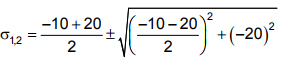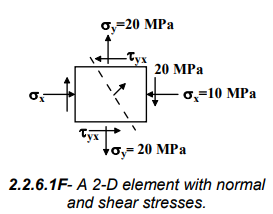This gives 20MPa and 30 MPa
The principal planes are given by tan2 θ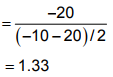The two values are 26.56 and 116.56o

The oriented element to show the principal stresses is shown in figure-2.2.6.2.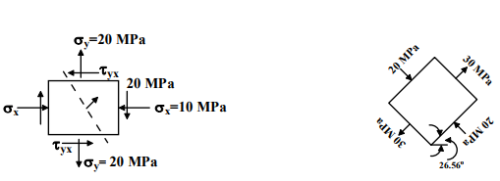2.2.6.2F- Orientation of the loaded element in the left to show the principal stresses.

Offer running on EduRev: Apply code STAYHOME200 to get INR 200 off on our premium plan EduRev Infinity!

## Machine Design

57 videos|71 docs|102 tests

,

,

,

,

,

,

,

,

,

,

,

,

,

,

,

,

,

,

,

,

,

;